Next: MAXIMUM QUADRATIC ASSIGNMENT Up: Routing Problems Previous: MINIMUM RECTILINEAR GLOBAL ROUTING   Index

### MINIMUM TRAVELING REPAIRMAN

• INSTANCE: Graph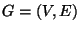, start vertex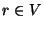, length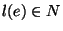for each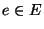satisfying the triangle inequality.

• SOLUTION: A walk starting in r visiting all vertices in G, i.e., a permutation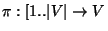such that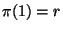, where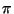describes in which order the vertices are visited for the first time.

• MEASURE:, whereis the total distance traversed in the walk from r until we first visit v.

• Good News: Approximable within 10.78 .

• Comment: Also called the Minimum Latency Problem. For fixed-dimensional Euclidean spaces the problem is approximable within 3.59  and admits a quasi polynomial PTAS . Variation in which the objective is the maximum search ratio, i.e.,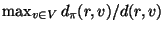is approximable within 6 and is APX-complete . Variation in which the graph metric is used (all lengths 1 or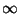) is approximable within 1.662 .

Viggo Kann
2000-03-20